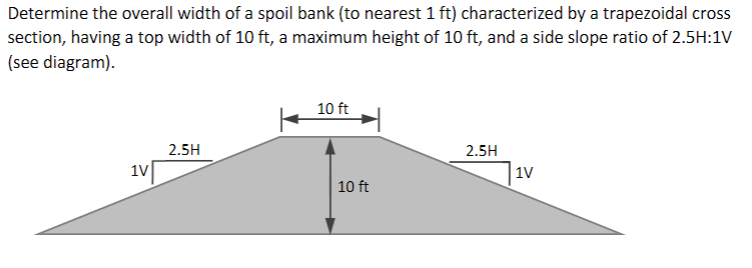Home / Expert Answers / Civil Engineering / determine-the-overall-width-of-a-spoil-bank-to-nearest-1-mathrm-ft-characterized-by-a-t-pa117

# (Solved): Determine the overall width of a spoil bank (to nearest $$1 \mathrm{ft}$$ ) characterized by a t ...Determine the overall width of a spoil bank (to nearest $$1 \mathrm{ft}$$ ) characterized by a trapezoidal cross section, having a top width of $$10 \mathrm{ft}$$, a maximum height of $$10 \mathrm{ft}$$, and a side slope ratio of $$2.5 \mathrm{H}: 1 \mathrm{~V}$$ (see diagram).

We have an Answer from Expert